# Difference Between Heat engine,Refrigerator and Heat Pump

Heat Engine

In a heat engine the heat supplied to the engine is converted into useful work. If  Q2 is the heat supplied to the engine and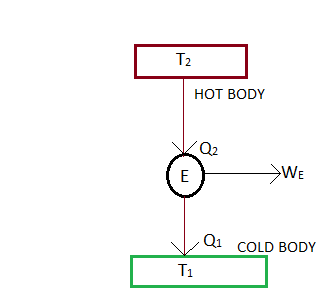Q1  is the heat rejected from the engine , then the net work done by the engine is given by

W E =Q2 –Q1

The performance of a heat engine is expressed by it’s efficiency . We know that the efficiency or coefficient of performance of an engine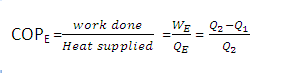Where –    WE = Work done by engine
Ta = Atmospheric temperature

Refrigerator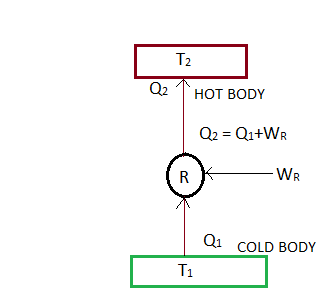Refrigerator is a reversed heat engine which either cool or maintain the temp. of a body (T1) lower than the atmospheric temp. (Ta) .  This is done by extracting heat (Q1) from a cold body and delivering it to a hot body (Q2). In doing so work WR is required to be done on the system . According to first law of thermodynamics      WR= Q2-Q1

The performance of refrigerator is expressed  by the ratio of heat taken from the cold body (Q1) to the amount of work required to be done on the system (WR) . This ratio is called coefficient of  performance .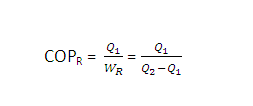Heat Pump

Heat pump extracts heat (Q1) from a cold body and delivers it to a hot body . Thus, there is no difference between the cycle of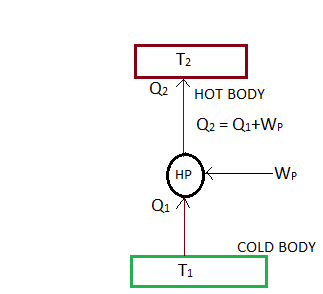operation of  a heat pump and a refrigerator . The main difference between the two is in their operating temp.  A refrigerator works between the cold body  temp. (T1) and the atmospheric temp. (Ta) whereas the heat pump operates between the hot body temp. (T2) and the atmospheric temp. (Ta) . Refrigerator used for cooling in summer and heat pump used for heating in winter season.

WP= Q2– Q1

The COP of heat pump is expressed by the ratio of the amount of heat delivered  to the hot body (Q2) to the amount of work required to be done on the system.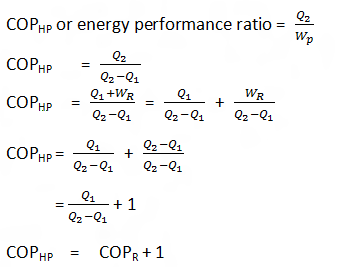From above we see that the COP may be less than one or greater than one depending on the type of refrigeration system used . But the COP of a heat  pump is always greater than one .

[ssba]

This site uses Akismet to reduce spam. Learn how your comment data is processed.